Important Update
The Guide Feature will be discontinued after December 15th, 2023. Until then, you can continue to access and refer to the existing guides.Gaurav Singhal

# Transfer Learning in Deep Learning Using Tensorflow 2.0

• Nov 16, 2020
• 8,768 Views
• Nov 16, 2020
• 8,768 Views

## Overview

TensorFlow is one of the top deep learning libraries today. A previously published guide, Transfer Learning with ResNet, explored the Pytorch framework. This guide will take on transfer learning (TL) using the TensorFlow library. The TensorFlow framework is smooth and uncomplicated for building models.

In this guide, you will learn how to use a pre-trained MobileNet model using TensorFlow Hub (TFHub), a library for the publication, discovery, and consumption of reusable parts of machine learning models.

## Why MobileNet?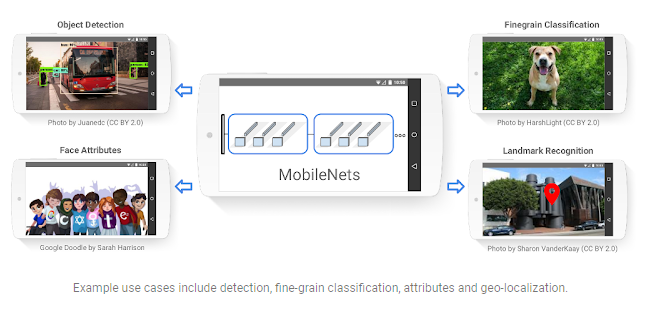Source: Google AI Labs

Computer vision networks have the responsibility to make a deeper network achieve higher accuracy. In short, the deeper the model, the harder it is to optimize. For compact applications, it becomes inconvenient to maintain the number of operations as the system has limited computation and power.

MobileNet was introduced to mitigate these problems. It has two versions, MobileNet-V1 and MobileNet-V2. This guide will stick to MobileNet-V2. Compared to other models, such as Inception, MobileNet outperforms with latency, size, and accuracy. To build lighter deep neural networks, it uses Depthwise Separable Convolution (DSC) layers.

## MobileNet Architecture

The main aim of TL is to implement a model quickly. There will be no change in the MobileNet architecture whatsoever. The model will transfer the features it has learned from a different dataset that has performed the same task.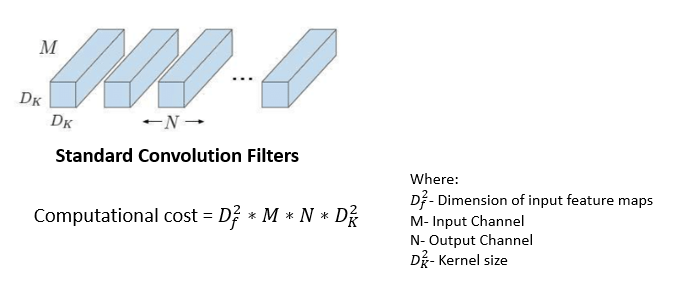Source: Thermal Stresses—Advanced Theory and Applications

While applying the composite function in the standard convolution layer, the convolution kernel is applied to all the channels of the input image and slides the weighted sum to the next pixel. MobileNet uses this standard convolution filter on only the first layer.

### Depthwise Separable Convolution

The next layer is depthwise separable convolution, which is the combination of depthwise and pointwise convolution.

#### Depthwise Convolution

Unlike standard convolution, a depthwise convolution maps only one convolution on each input channel separately. The channel dimension of the output image (3 RGB) will be the same as that of an input image.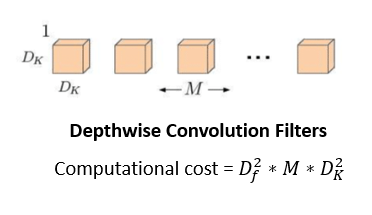#### Pointwise Convolution

This is the last operation of the filtering stage. It's more or less similar to regular convolution but with a 1x1 filter. The idea behind pointwise convolution is to merge the features created by depthwise convolution, which creates new features.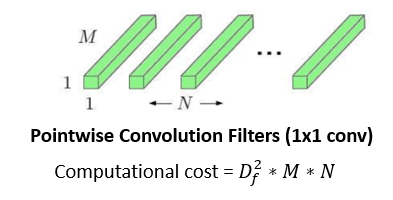The cost function of DSC is the sum of the cost of depthwise and pointwise convolution.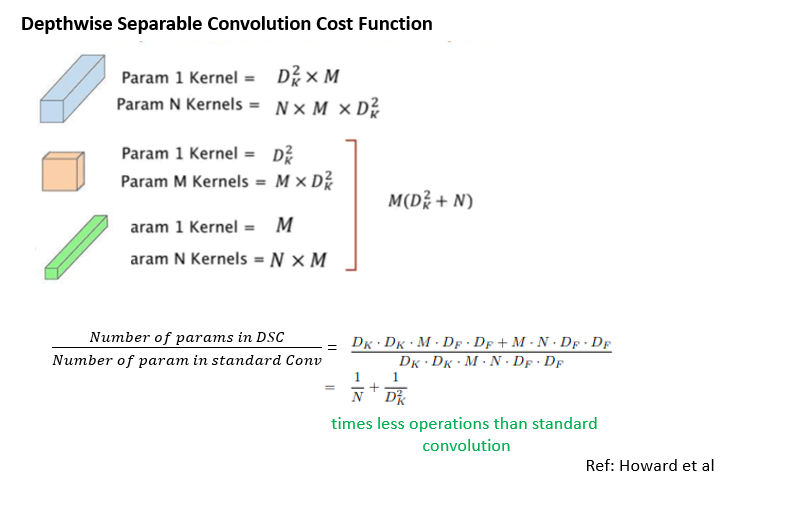Source: MobileNets: Efficient Convolutional Neural Networks for Mobile Vision Applications

Other than this, MobileNet offers two more parameters to reduce the operations further:

• Width Multiplier: This introduces the variable α ∈ (0, 1) to thin the number of channels. Instead of producing N channels, it will produce αxN channels. It will choose 1 if you need a smaller model.
• Resolution Multiplier: This introduces the variable ρ ∈ (0, 1), it is used to reduce the size of the input image from 244, 192, 160px or 128px. 1 is the baseline for image size 224px.

You can train the model on a 224x224 image and then use it on 128x128 images as MobileNet uses Global Average Pooling and doesn't flatten layers.

### MobileNet-V2

The MobileNet-V2 pre-trained version is available here. Its weights were initially obtained by training on the ILSVRC-2012-CLS dataset for image classification (Imagenet).

The basic building blocks in MobileNet-V1 and V2: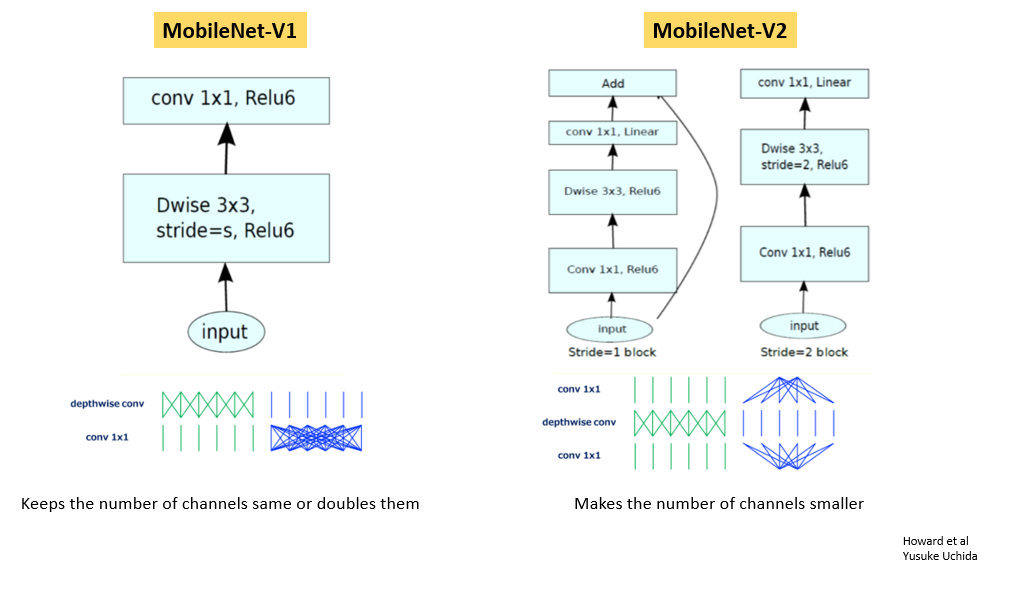Source: MobileNets: Efficient Convolutional Neural Networks for Mobile Vision Applications

The final MobileNet-V2architecture looks like this: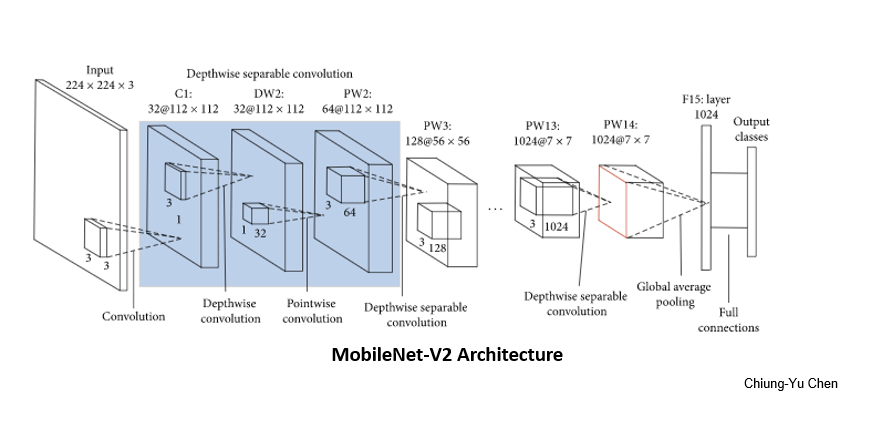Source: References 3

## Build a MobileNet Model

Now that you know the elements of MobileNet, you can build the model from scratch to make it more customize or use the pre-trained-model and save some time.

Let's see how the code works. Start with importing the essential libraries.

``````1import os
2import tensorflow as tf
3import tensorflow_hub as hub
4from tensorflow.keras import layers, Sequential
5import tensorflow.keras.backend as K
6from tensorflow.keras.preprocessing import image
7from tensorflow.keras.preprocessing.image import ImageDataGenerator
8from tensorflow.keras.callbacks import EarlyStopping
9import pathlib
10import matplotlib.pyplot as plt
11import seaborn as sns
12sns.set()
13import numpy as np``````
python
``````1train_root = "../alien-vs-predator-images/train"
2test_root = "../alien-vs-predator-images/validation"``````
python
``````1image_path = train_root + "/alien/103.jpg"
4    image_rel = pathlib.Path(image_path).relative_to(train_root)
5    print(image_rel)
python

Testing the above function:

``1image_load(image_path)``
python

Output: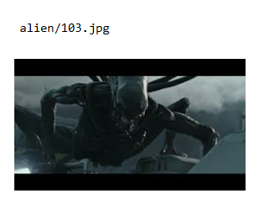The data seems ready. But to use it, you need load it into the model.

``````1train_generator = ImageDataGenerator(rescale=1/255)
2test_generator = ImageDataGenerator(rescale=1/255)
3
4train_image_data = train_generator.flow_from_directory(str(train_root),target_size=(224,224))
5test_image_data = test_generator.flow_from_directory(str(test_root), target_size=(224,224))``````
python

Output:TFHub also distributes models without the top classification layer. It uses `feature_extractor` for transfer learning.

``````1# Model-url
python
``````1def feature_extractor(x):
2  feature_extractor_module = hub.Module(feature_extractor_url)
3  return feature_extractor_module(x)
4
5IMAGE_SIZE = hub.get_expected_image_size(hub.Module(feature_extractor_url))
6IMAGE_SIZE``````
python

Output:``````1for image_batch, label_batch in train_image_data:
2    print("Image-batch-shape:",image_batch.shape)
3    print("Label-batch-shape:",label_batch.shape)
4    break``````
python

Output:``````1for test_image_batch, test_label_batch in test_image_data:
2    print("Image-batch-shape:",test_image_batch.shape)
3    print("Label-batch-shape:",test_label_batch.shape)
4    break``````
python

Output:``1feature_extractor_layer = layers.Lambda(feature_extractor,input_shape=IMAGE_SIZE+)``
python

Freeze the variables in the feature extractor so that training only modifies the new classifier layer.

``1feature_extractor_layer.trainable = False``
python
``````1model = Sequential([
2    feature_extractor_layer,
3    layers.Dense(train_image_data.num_classes, activation = "softmax")
4    ])
5model.summary()``````
python

Output:Next, initialize the TFHub module.

``````1sess = K.get_session()
2init = tf.global_variables_initializer()
3
4sess.run(init)``````
python

Test a single image.

``````1result = model.predict(image_batch)
2result.shape``````
python
``1(32, 2)``

The next step is to compile the model with an optimizer.

``````1model.compile(
3    loss = "categorical_crossentropy",
4    metrics = ['accuracy']
5    )``````
python

Create a custom callback to visualize the training progress during every epoch.

``````1class CollectBatchStats(tf.keras.callbacks.Callback):
2  def __init__(self):
3    self.batch_losses = []
4    self.batch_acc = []
5
6  def on_batch_end(self, batch, logs=None):
7    self.batch_losses.append(logs['loss'])
8    self.batch_acc.append(logs['acc'])
9
10# Early stopping to stop the training if loss start to increase. It also avoids overfitting.
11es = EarlyStopping(patience=2,monitor="val_loss")``````
python

Then, use CallBacks to record accuracy and loss.

``````1batch_stats = CollectBatchStats()
2# fitting the model
3model.fit((item for item in train_image_data), epochs = 3,
4         steps_per_epoch=21,
5         callbacks = [batch_stats, es],validation_data=test_image_data)``````
python

Output:Now, get the ordered list of labels.

``````1label_names = sorted(train_image_data.class_indices.items(), key=lambda pair:pair)
2label_names = np.array([key.title() for key, value in label_names])
3label_names``````
python

Output:You're almost finished. Run predictions for the test batch.

``````1result_batch = model.predict(test_image_batch)
2
3labels_batch = label_names[np.argmax(result_batch, axis=-1)]
4labels_batch``````
python

Output:And finally, show the predicted results.

``````1plt.figure(figsize=(13,10))
2for n in range(30):
3  plt.subplot(6,5,n+1)
4  plt.imshow(test_image_batch[n])
5  plt.title(labels_batch[n])
6  plt.axis('off')
7  plt.suptitle("Model predictions")``````
python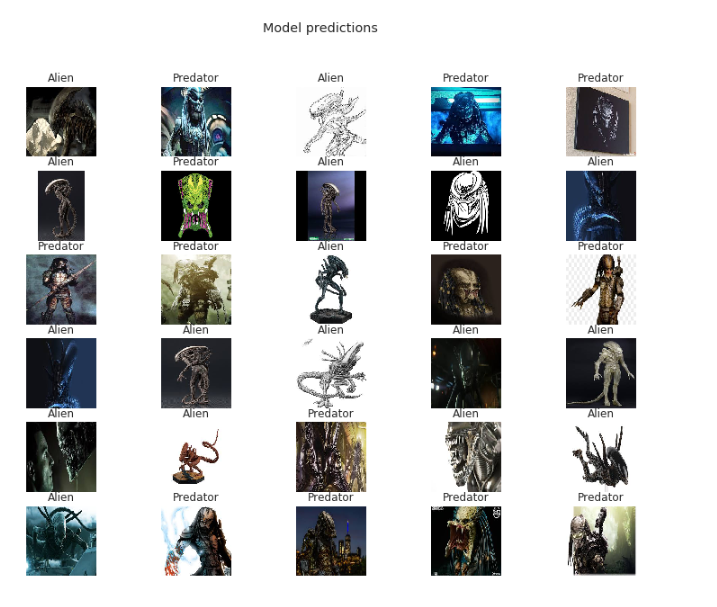You may save the model for later use.

## Conclusion

Well done! The accuracy is ~94%. Your small but powerful NN model is ready. The trade-off between performance and speed is acceptable unless it is deployable on an embedded device and gives real-time offline detection.

To have good control of building the models, I recommend running the code on different datasets. Notice the accuracy and run time. This guide has given you a brief explanation of how to use pre-trained models in the TensorFlow library and MobileNet architecture. Read the links mentioned in the guide for a better understanding. You can create compact and insanely fast classifiers using MobileNets. They are widely used in NLP applications.

If you have any questions, feel free to reach out to me at CodeAlphabet.